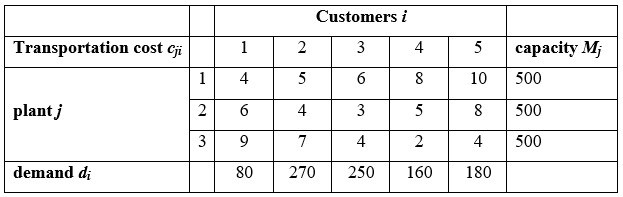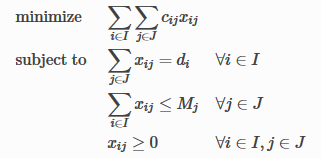# Optimization Modelling

## Overview

In the past, we used to model a real-world optimization problem then solve it with an optimization solver and give the optimal result to managers and decision makers. Thus, optimization models were traditionally designed for use in strategic/tactical decisions rather than operational ones.

These days, however, many in industry want to plan and make optimal decisions regularly as a part of their hourly, daily, or weekly operations.

PuLP is arguably the easier module to learn from the three (PuLP, SciPy, Pyomo), however it can deal only with linear optimization problems. Pyomo seems to be more supported than PuLP, has support for nonlinear optimization problems, and last but not the least, can do multi-objective optimization.

## Typical situation in real life

Let’s consider simplified transportation type problem. We have set of customers $I = [1,2,3,4,5]$ and set of factories $J = [1,2,3]$. Each customer has some fixed product demand $d_i$ and each factory has fixed production capacity $M_j$. There are also fixed transportation costs to deliver one unit of good from factory $j$ to customer $i$.Our task is to deliver necessary amount of goods to each customer (satisfy customer demand and factories production capacity) at minimal total transportation cost.

Mathematically this optimization problem can be described as follows.To formulate this situation as optimization problem we must separate it into 3 main components:

• decision variables — quantities of goods to be sent from factory j to customer i (positive real numbers)
• constraints — total amount of goods must satisfy both customer demand and factory production capacity (equalities/inequalities that have linear expression on the left-hand side)
• objective function — find such values of decision variables that total transportation cost is the lowest (linear expression in this case)

## My own experience

I encountered an interesting question from playing a game. I want to find the optimized combination of 3 types of battleships in a fleet which will satisfy these conditions:

• quantity of ship type 1: $x <= 8$
• quantity of ship type 2: $y <= 12$
• quantity of ship type 3: $z <= 12$
• the max unit supply must be equal or less than 300: $18*x + 18*y + 16*z <= 300$
• the output damage from this combinations is max: $22013*x + 12653*y + 10312*z$

Find below a solution using PuLP in GitHub Gist.